# A curva normal

• Nota de Estudos
• Rever Tópicos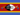Samkeliso R S. 0 0 not clear either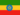Henock A. 0 0 done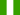Juliet N. 0 0 not very clear on this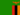Edson C. 0 0 A normal distribution has a bell-shaped curve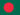Jahidul I. 0 0 the normal (or Gaussian) distribution is a very common continuous probability distribution. Normal distributions are important in statistics and are often used in the natural and social sciences to represent real-valued random variables whose distributions are not known. A series of tests was given to a large number of students and the results of these tests were combined to obtain a Verbal Aptitude score for each student. For the whole group it was found that: Mean = 595, and Standard Deviation = 73. Samples of increasing size (200, 500, 1000, and 5000) were taken and the scores for each sample graphed as a histogram. As the sample size increased the distribution became more symmetrical and approached the shape of the normal curve.Jahidul I. 0 0 what is curve ?
• Text Version### Você recebeu uma nova notificação

Clique aqui para visualizar todos eles